• ## kmeans++

千次阅读 2016-07-03 10:07:09
kmeans++是kmean算法的改进，原来kmean算法在原始数据的最小、最大区间内均等的选择K个聚类中心，然而kmeans++却是从原始数据中选择K个作为初始聚类中心，这种思路的效果优于kmean.下面是kmeas++的matlab代码，后面...
kmeans++是kmean算法的改进，原来kmean算法在原始数据的最小、最大区间内均等的选择K个聚类中心，然而kmeans++却是从原始数据中选择K个作为初始聚类中心，这种思路的效果优于kmean.下面是kmeas++的matlab代码，后面实例中使用的Gauss_sample函数是我的上一篇博文。
1.函数
function [K_center,seed]=kmeans_plus(data,K,LOOP) %此代码对应kmeans++算法，该算法是对kmeans的改进，性能优于kmeans % data是待分类的数据,尺寸为 (dim*N)，dim代表每个样本的维数，N代表样本个数 % K 是设定的聚类数量，即把N个样本分成K类，每一类内部的差别小，各类之间的差别大  %首先，确定K个初始聚类中心，这些聚类中心均来源于原始的N个样本 [dim,N]=size(data); K_center=zeros(dim,K);  seed=[]; str=round(rand(1,1)*N); seed=[seed,data(:,str)];  %随机选择的第一个聚类中心 for k=2:1:K     num_seed=size(seed,2);     Dis_temp=zeros(k,N);     d_min=zeros(1,N);     for i=1:1:N         d_min(1,i)=(seed(:,1)-data(:,i))'*(seed(:,1)-data(:,i));         for j=1:1:num_seed             Dis_temp(j,i)=(seed(:,j)-data(:,i))'*(seed(:,j)-data(:,i));             if d_min(1,i)>Dis_temp(j,i)                 d_min(1,i)=Dis_temp(j,i);             end         end     end     pos=find(d_min==max(d_min));     seed=[seed,data(:,pos)]; end  K_center=seed; %所有的K个初始聚类中心已经确定，下面按照传统的kmeans算法执行 for loop=1:1:LOOP DIS=zeros(K,N);  %计算N个样本与K个聚类之间的距离，行代表聚类，列代表样本 for i=1:1:N     for j=1:1:K         d=K_center(:,j)-data(:,i);         DIS(j,i)=d'*d;     end end  class=zeros(1,N); for i=1:1:N     class(1,i)=1;     d=DIS(1,i);     for j=1:1:K         if DIS(j,i)<d             d=DIS(j,i);             class(1,i)=j;         end     end end  for k=1:1:K     sum=zeros(dim,1);     idx=find(class==k);     count=length(idx);     for c=1:1:count         sum=sum+data(:,idx(c));     end K_center(:,k)=sum/count; end  end

2.实例
[data,w,m,s]=Gauss_sample(2,5,100); figure(1);clf plot(data(1,:),data(2,:),'b*');  [K_center,seed]=kmeans_plus(data,5,100);

原始高斯分布的聚类中心 m=
-163   261    26  -115   157   -196    97   -97    55  -169
经过kmeans++算法计算得到的聚类中心 K_center=
263.1232 -164.0305 -114.1521  157.6263   27.8879    95.3258 -195.5066   55.2112 -168.8433  -95.0808  经过一一比对，效果还不错，下面用一幅图显示结果，蓝色星星代表数据，红色圆圈是kmeans++找到的聚类中心：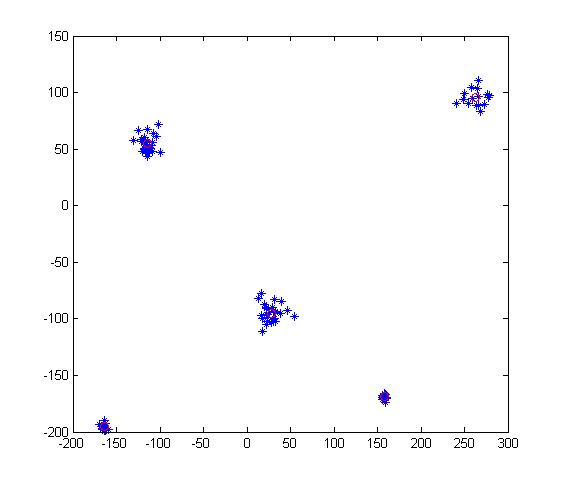展开全文• Kmeans、Kmeans++和KNN算法比较.pdf
• ***题记：*我一直在路上，害怕停下 在我的另一篇博客里《读sklearn源码学机器学习——kmeans聚类算法》我详细的阐述了kmeans算法的工作过程。截至目前为止，还没有深入的刨析kmeans算法的工作原理(会用...kmeans++是D
***题记：*我一直在路上，害怕停下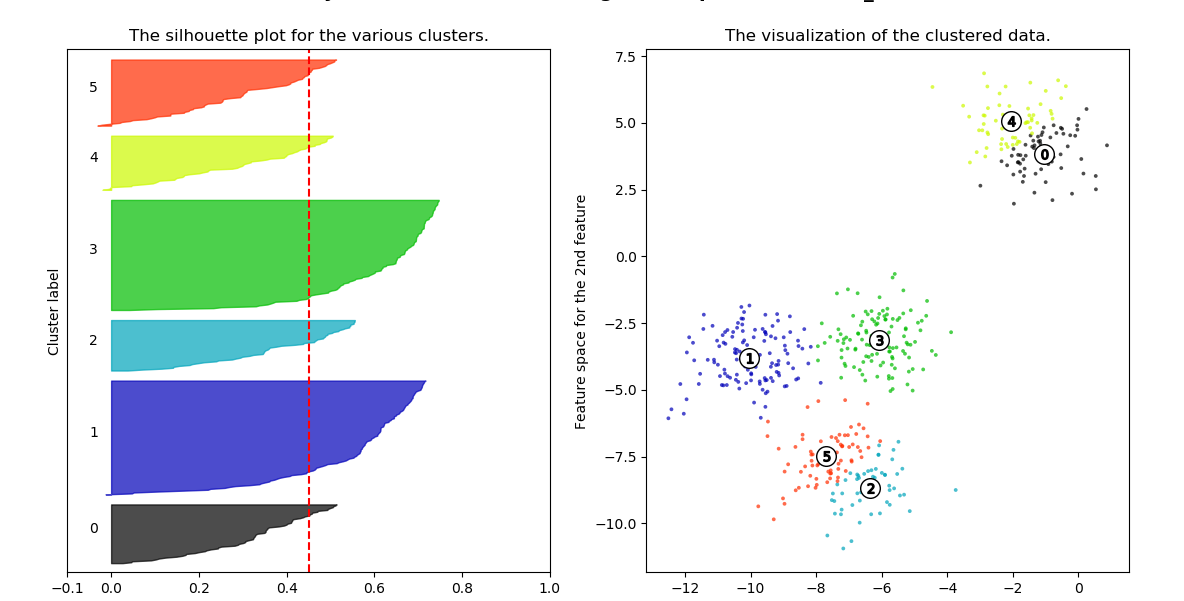在我的另一篇博客里《读sklearn源码学机器学习——kmeans聚类算法》我详细的阐述了kmeans算法的工作过程。截至目前为止，还没有深入的刨析kmeans算法的工作原理(会用和知道怎么用，跟理解背后深刻的数学原理是有本质区别的，我对此深感敬畏)。其实kmeas算法和高斯混合算法都是em算法的具体应用。今天站在工程应用的角度，刨析kmeans在工程中的应用和优化。主要是弥补我上篇博客中没有说明白的两个函数（elativeDist,squaredNorna）和一个初始化。
1、kmeans++算法
kmeans++是David Arthur and Sergei Vassilvitskii在一篇名为“k-means++: The Advantages of Careful Seeding（http://ilpubs.stanford.edu:8090/778/1/2006-13.pdf）文中中首次提及的，可以用来解决kmeans在初始化的时候，随机选取初始点带来的负面影响。算法的工作过程如下：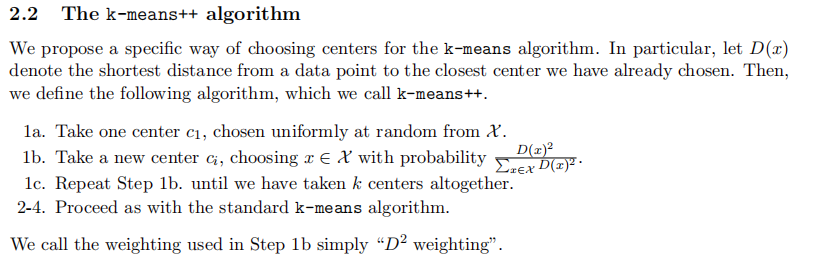翻译成中文： 1a、随机的选取一个样本点作为一个一个聚类中心。 2b、在选择下一个聚类中心的时候，以到达现有聚类中心的距离为依据进行聚类中心得选择。离现在的聚类中心越远被选择的概率越大。 （一般通过轮盘赌选择，概率一般用欧式距离平方占比） 1c、重复上述的过程直到选出所有的聚类中心。 基本依据：在选择初始距离的时候，样本之间离得越远越好。 kmeans++实现过程： 数据通过矩阵的形式存在matrix_data中（个人觉得自己的实现过程还是优雅且美妙的）。 （可以仔细体会下对轮盘赌的实现过程以及numpy相应函数的应用，可以猜测np.searchsorted()的算法复杂度最高时log(n),比我们遍历选取要划算多了）
center=np.zeros((n_cluster,n_feature))
center=matrix_data[np.random.randint(n_samples)]
for i in range(1,n_cluster):
#计算每个样本点到已有中心点的距离
distance_to_centers=euclideanDistance(matrix_data,center[[i for i in range(i)]],square=True)
#选取据距离最小值
closed_distance=np.min(distance_to_centers,axis=1)
#轮盘赌
denominator=closed_distance.sum()
point=np.random.rand()*denominator#轮盘赌的指针
be_choosed=np.searchsorted(np.cumsum(closed_distance),point)
be_choosed=min(be_choosed,n_samples-1)#避免选的是最后一个而造成下标越界
center[i]=matrix_data[be_choosed]

其中欧式距离的计算函数euclideanDistance实现原理在上一篇博客中证明过，过程如下：
import pandas as pd
import numpy as np
def rowNorms(X):#对行每个元素取平方加和
return np.einsum("ij,ij->i",X,X)
def euclideanDistance(x,y,square=False):#x的每个样本与y之间的距离
"""
:param x: 矩阵x
:param y: 矩阵y
:param squared: 表示是否返回二者欧式距离的平方值,很明显如果返回欧式距离的平方，计算量又小一些
:return: 矩阵x中的每一个样本与y中样本之间的距离
"""
"""
对于这个操作的理解一定要理解下面这个操作
np.array([,,])+np.array([[1,2,3],[4,5,6],[7,8,9]])
Out:
array([[ 2,  3,  4],
[ 6,  7,  8],
[ 8,  9, 10]])
np.array([[ 2,  3,  4],[ 6,  7,  8],[ 8,  9, 10]])+np.array([1,2,3])
Out:
array([[ 3,  5,  7],
[ 7,  9, 11],
[ 9, 11, 13]])
P矩阵与C矩阵每一行之间的距离可以用公式[[p1^2],[p2^2],[...]]-2PC^T+[c1^2,c2^2,c3^2...]
(p1^2是p1行向量平方和，c1^2是c1行向量平方和)
"""
xx=rowNorms(x)[:,np.newaxis]#转化为列向量，便于让dot的每一行都相加同一个数
yy=rowNorms(y)[np.newaxis,:]#与xx同理
dot=np.dot(x,y.T)
res = xx + yy - 2 * dot
return res if square else np.sqrt(res)

kmeans++的优化
该方法David Arthur and Sergei Vassilvitskii没有发表出来，但是在机器学习开源社区上被用到，即在用kmeas++计算距离的过程中，每一轮再增加一个最大迭代次数，比如通过轮盘赌一次选取n_local_trials个，然后再依次选取能做到使距离平方和最小的聚类中心点（总距离平方和最小中的离现有中心点最大的点）。n_local_trals的选取原则为（2+log(聚类中心点数)）。 实现过程如下（引用的sklearn开源社区源码（https://github.com/scikit-learn/scikit-learn））：
n_samples, n_features = X.shape
centers = np.empty((n_clusters, n_features), dtype=X.dtype)
n_local_trials = 2 + int(np.log(n_clusters))
center_id = random_state.randint(n_samples)
centers = X[center_id]
closest_dist_sq = euclidean_distances(
centers[0, np.newaxis], X, Y_norm_squared=x_squared_norms,
squared=True)
current_pot = closest_dist_sq.sum()
for c in range(1, n_clusters):
rand_vals = random_state.random_sample(n_local_trials) * current_pot
candidate_ids = np.searchsorted(stable_cumsum(closest_dist_sq),
rand_vals)
np.clip(candidate_ids, None, closest_dist_sq.size - 1,
out=candidate_ids)

distance_to_candidates = euclidean_distances(
X[candidate_ids], X, Y_norm_squared=x_squared_norms, squared=True)
np.minimum(closest_dist_sq, distance_to_candidates,
out=distance_to_candidates)
candidates_pot = distance_to_candidates.sum(axis=1)
best_candidate = np.argmin(candidates_pot)
current_pot = candidates_pot[best_candidate]
closest_dist_sq = distance_to_candidates[best_candidate]
best_candidate = candidate_ids[best_candidate]
centers[c] = X[best_candidate]

return centers


距离计算的优化
假设有4个样本3个特征如下：
_f1f2f31123245637894101112
再比如想要把样本聚类为2类，这样类的聚类中线为1和2。将上面数据存为矩阵： x=np.array([[1,2,3],[4,5,6],[7,8,9],[10,11,12]]) 通过euclideanDistance计算所有样本点到两个聚类中心的距离，如下：
euclideanDistance(x,x[[0,1]])
Out:
array([[ 0.        ,  5.19615242],
[ 5.19615242,  0.        ],
[10.39230485,  5.19615242],
[15.58845727, 10.39230485]])

结果显示1到样本1的距离0，到样本2的距离为5.19615242，2到样本1的距离为5.19615242到样本2的距离为0，依次类推我们就得到了各个样本到各个聚类中心的距离，并以此将各个样本贴上不同的类别标签上面的类别标签为[1,2,2,2]。 所以我们再选择的过程中直接比较的是行向量的相对大小，那如果我们在求||x-x[[0,1]]||的时候行向量同时减去一个||x||^2哪？分类的结果其实是不变的，但是对于这里的距离求取过程却可以简化为如下：
def relativeDist(x,y):#x的每个样本与y之间的相对距离
"""
我们知道，如果单纯选出x行向量与y之间最小的距离，完全可以同时减去xx
也就是(x-y)^2-x^2
"""
yy=rowNorms(y)[np.newaxis,:]
dot=np.dot(x,y.T)
res=-2*dot+yy
return res

该方法在计算距离的时候每次减少的计算量与x的大小成正比，减少了计算量。 上面例子用relativeDist求取的结果为：
relativeDist(x,x[[0,1]])
Out:
array([[ -14,   13],
[ -50,  -77],
[ -86, -167],
[-122, -257]])

分类结果依然是[1,2,2,2]。
展开全文聚类 python 算法 机器学习
• scikit-learn kmeans++.pdf
• Kmeans算法的缺陷 • 聚类中心的个数K 需要事先给定，但在实际中这个 K 值的选定是非常难以估计的，很多时候，事先并不知道给定的数据集应该分成多少个类别才最合适 • Kmeans需要人为地确定...（可以使用Kmeans+
 转载：http://www.cnblogs.com/zjiaxing/p/5616679.html
Kmeans算法的缺陷
• 聚类中心的个数K 需要事先给定，但在实际中这个 K 值的选定是非常难以估计的，很多时候，事先并不知道给定的数据集应该分成多少个类别才最合适 • Kmeans需要人为地确定初始聚类中心，不同的初始聚类中心可能导致完全不同的聚类结果。（可以使用Kmeans++算法来解决）
K-Means ++ 算法
k-means++算法选择初始seeds的基本思想就是：初始的聚类中心之间的相互距离要尽可能的远。
1. 从输入的数据点集合中随机选择一个点作为第一个聚类中心 2. 对于数据集中的每一个点x，计算它与最近聚类中心(指已选择的聚类中心)的距离D(x) 3. 选择一个新的数据点作为新的聚类中心，选择的原则是：D(x)较大的点，被选取作为聚类中心的概率较大 4. 重复2和3直到k个聚类中心被选出来 5. 利用这k个初始的聚类中心来运行标准的k-means算法
从上面的算法描述上可以看到，算法的关键是第3步，如何将D(x)反映到点被选择的概率上，一种算法如下：
1. 先从我们的数据库随机挑个随机点当“种子点” 2. 对于每个点，我们都计算其和最近的一个“种子点”的距离D(x)并保存在一个数组里，然后把这些距离加起来得到Sum(D(x))。 3. 然后，再取一个随机值，用权重的方式来取计算下一个“种子点”。这个算法的实现是，先取一个能落在Sum(D(x))中的随机值Random，然后用Random -= D(x)，直到其<=0，此时的点就是下一个“种子点”。 4. 重复2和3直到k个聚类中心被选出来 5. 利用这k个初始的聚类中心来运行标准的k-means算法
可以看到算法的第三步选取新中心的方法，这样就能保证距离D(x)较大的点，会被选出来作为聚类中心了。至于为什么原因比较简单，如下图所示：假设A、B、C、D的D(x)如上图所示，当算法取值Sum(D(x))*random时，该值会以较大的概率落入D(x)较大的区间内，所以对应的点会以较大的概率被选中作为新的聚类中心。
展开全文• 原型聚类1.1 KMeans1.1.1 最小化成本函数1.1.2 实例1.2 KMeans++1.2.1 KMeans++ 初始化实例参考资料 相关文章： 机器学习 | 目录 机器学习 | 聚类评估指标 机器学习 | 距离计算 无监督学习 | KMeans之Skleaen...


文章目录
1. 原型聚类1.1 KMeans1.1.1 最小化成本函数1.1.2 实例
1.2 KMeans++1.2.1 KMeans++ 初始化实例

2. 在线可视化 KMeans参考资料

相关文章：

机器学习 | 目录
机器学习 | 聚类评估指标
机器学习 | 距离计算
无监督学习 | KMeans之Sklearn实现：电影评分聚类
无监督学习 | 层次聚类 之凝聚聚类原理及Sklearn实现
无监督学习 | DBSCAN 原理及Sklearn实现
无监督学习 | GMM 高斯混合聚类原理及Sklearn实现
1. 原型聚类
原型聚类亦称“基于原型的聚类”（prototypr-based clustering）。此类算法假设聚类结构能通过一组原型刻画，在现实聚类任务重及其常用。通常情形下，算法先对原型进行初始化，然后对原型进行迭代更新求解。采用不同的原型表示、不同的求解方式，将产生不同的算法，如 KMeans、LVQ、高斯混合。下面介绍 KMeans 算法，我们将在下一篇文章中介绍高斯混合算法。

“原型”是指样本空间具有代表性的点

1.1 KMeans
给定样本集

D

=

x

1

,

x

2

,

⋯

,

x

m

D={x_1,x_2,\cdots,x_m}

，“$k$ 均值”（k-means）算法针对聚类所得簇划分

C

=

C

1

,

C

2

,

⋯

,

C

k

C={C_1,C_2,\cdots,C_k}

最小化平方误差（残差平方和

S

E

S_E

）：

E

=

∑

i

=

1

k

∑

x

∈

C

i

∥

x

−

μ

i

∥

2

2

(1)

E=\sum_{i=1}^k \sum_{x\in C_i}\|x-\mu_i\|_2^2 \tag{1}

其中

μ

i

=

1

∣

C

i

∣

∑

x

∈

C

i

x

\mu_i=\frac{1}{|C_i|}\sum_{x\in C_i}x

是簇

C

i

C_i

的均值向量。直观来看，式 (1) 在一定程度上刻画了簇内样本围绕簇均值向量的紧密程度，

E

E

值越小则簇内样本相似度越高。
1.1.1 最小化成本函数
最小化式 (1) 并不容易，找到它的最优解需考察样本集

D

D

所有可能的簇划分，这是一个 NP 难问题。因此，k均值算法采用了贪心策略，通过迭代优化来近似求解式 (2) 。算法流程如下图所示：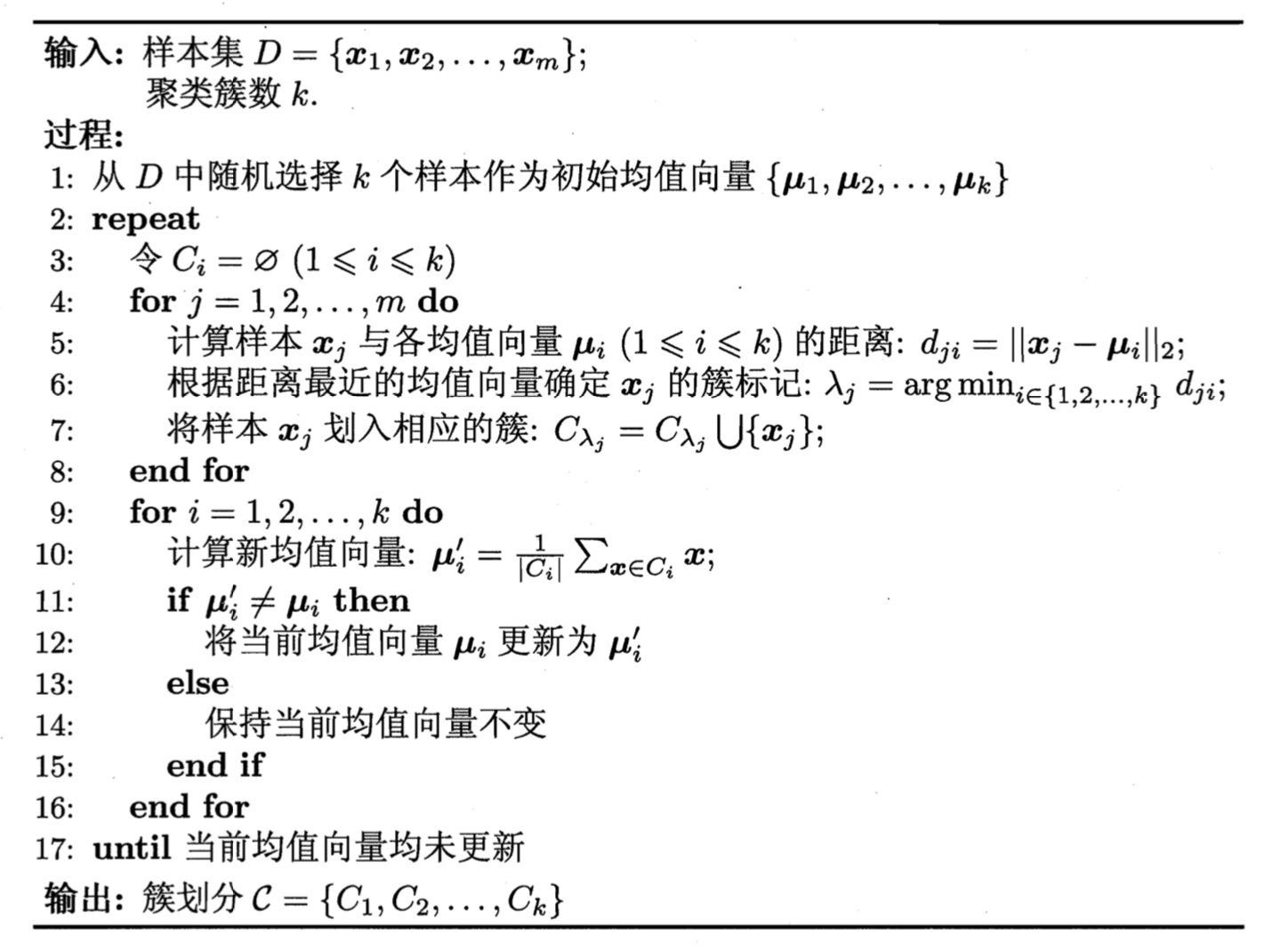图1 k 均值算法

其中第 1 行对均值向量进行初始化，在第 4-8 行对当前簇划分迭代更新，第 9-16 行对均值向量迭代更新，若迭代更新后均值结果保持不变，则在第 18 行对当前簇划分结果返回。

为避免运行时间过长，通常设置一个最大运行轮数（max_iter）或最小调整幅度阈值（tol），若达到最大轮数或调整幅度小于阈值，则停止运行。

1.1.2 实例
下面对西瓜数据集为例演示 k 均值算法的学习过程。为方便叙述，我们将变好为

i

i

的样本称为

x

i

x_i

，这是一个包含“密度”与“含糖率”两个属性值的二维向量。

表1 西瓜数据集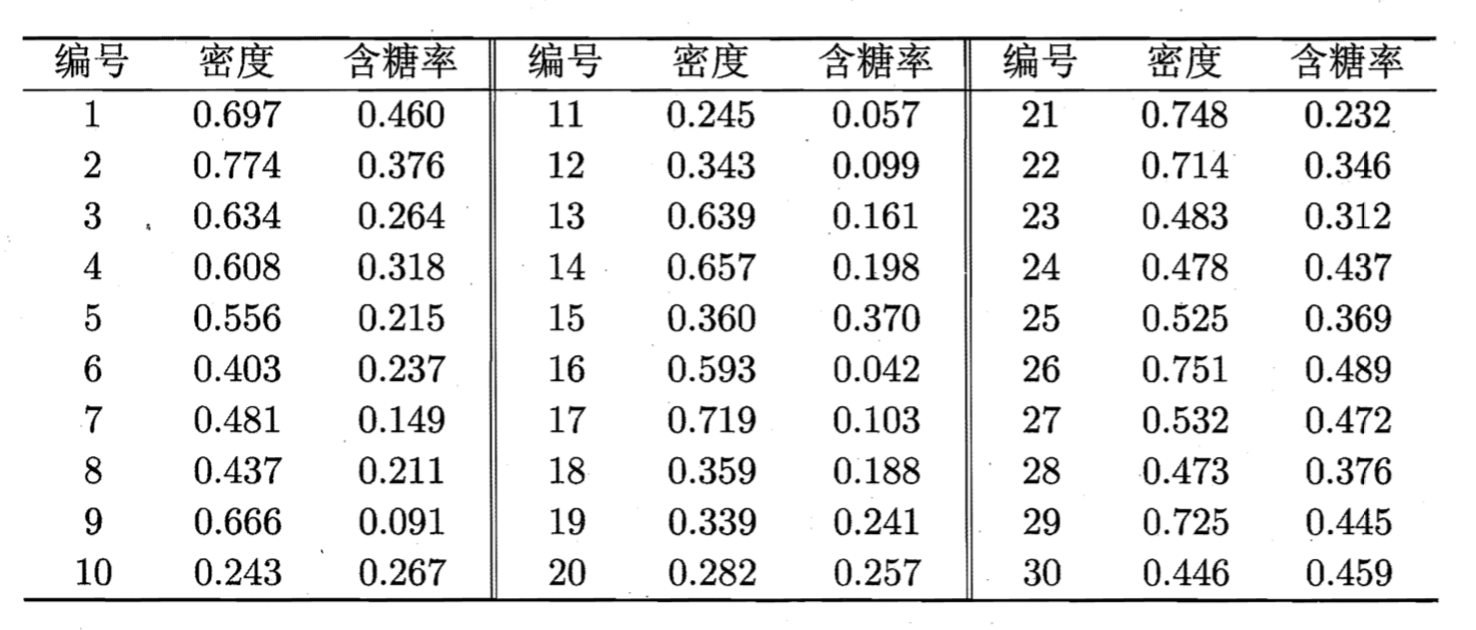假定聚类簇数（n_clusters）

k

=

3

k=3

，算法开始时随机选取三个样本

x

6

,

x

12

,

x

27

x_6,x_{12},x_{27}

作为初始均值向量，即：

μ

1

=

(

0.403

;

0.237

)

,

μ

2

=

(

0.343

;

0.0999

)

,

μ

3

=

(

0.532

;

0.472

)

\mu_1=(0.403;0.237),\mu_2=(0.343;0.0999),\mu_3=(0.532;0.472)

考察样本

x

1

=

(

0.697

;

0.460

)

x_1=(0.697;0.460)

，它与当前均值向量

μ

1

,

μ

2

,

μ

3

\mu_1,\mu_2,\mu_3

的距离分别为 0.369，0.506，0.166，因此

x

1

x_1

将划入簇

C

3

C_3

中。类似的，对数据集中的所有样本考虑一遍后，可得当前簇划分为：

C

1

=

{

x

5

,

x

6

,

x

7

,

x

8

,

x

9

,

x

10

,

x

13

,

x

14

,

x

15

,

x

17

,

x

18

,

x

19

,

x

20

,

x

23

}

;

C

2

=

{

x

11

,

x

12

,

x

16

}

;

C

3

=

{

x

1

,

x

2

,

x

3

,

x

4

,

x

21

,

x

22

,

x

24

,

x

25

,

x

26

,

x

27

,

x

28

,

x

29

,

x

30

;

}

\begin{aligned} C_1& =\{x_5,x_6,x_7,x_8,x_9,x_{10},x_{13},x_{14},x_{15},x_{17},x_{18},x_{19},x_{20},x_{23}\}; \\ C_2& = \{x_{11},x_{12},x_{16}\};\\ C_3& = \{x_{1},x_{2},x_{3},x_{4},x_{21},x_{22},x_{24},x_{25},x_{26},x_{27},x_{28},x_{29},x_{30};\} \\ \end{aligned}

于是，可从

C

1

、

C

2

、

C

3

C_1、C_2、C_3

分别求出新的均值向量：

μ

1

′

=

(

0.473

;

0.214

)

,

μ

2

′

=

(

0.394

;

0.066

)

,

μ

3

′

=

(

0.623

;

0.388

)

\boldsymbol{\mu}_{1}^{\prime}=(0.473 ; 0.214), \boldsymbol{\mu}_{2}^{\prime}=(0.394 ; 0.066), \boldsymbol{\mu}_{3}^{\prime}=(0.623 ; 0.388)

更新当前均值向量后，不断重复上述过程，如下图所示，第五轮迭代产生的结果与第四轮迭代相同，于是算法停止，得到最终的簇划分：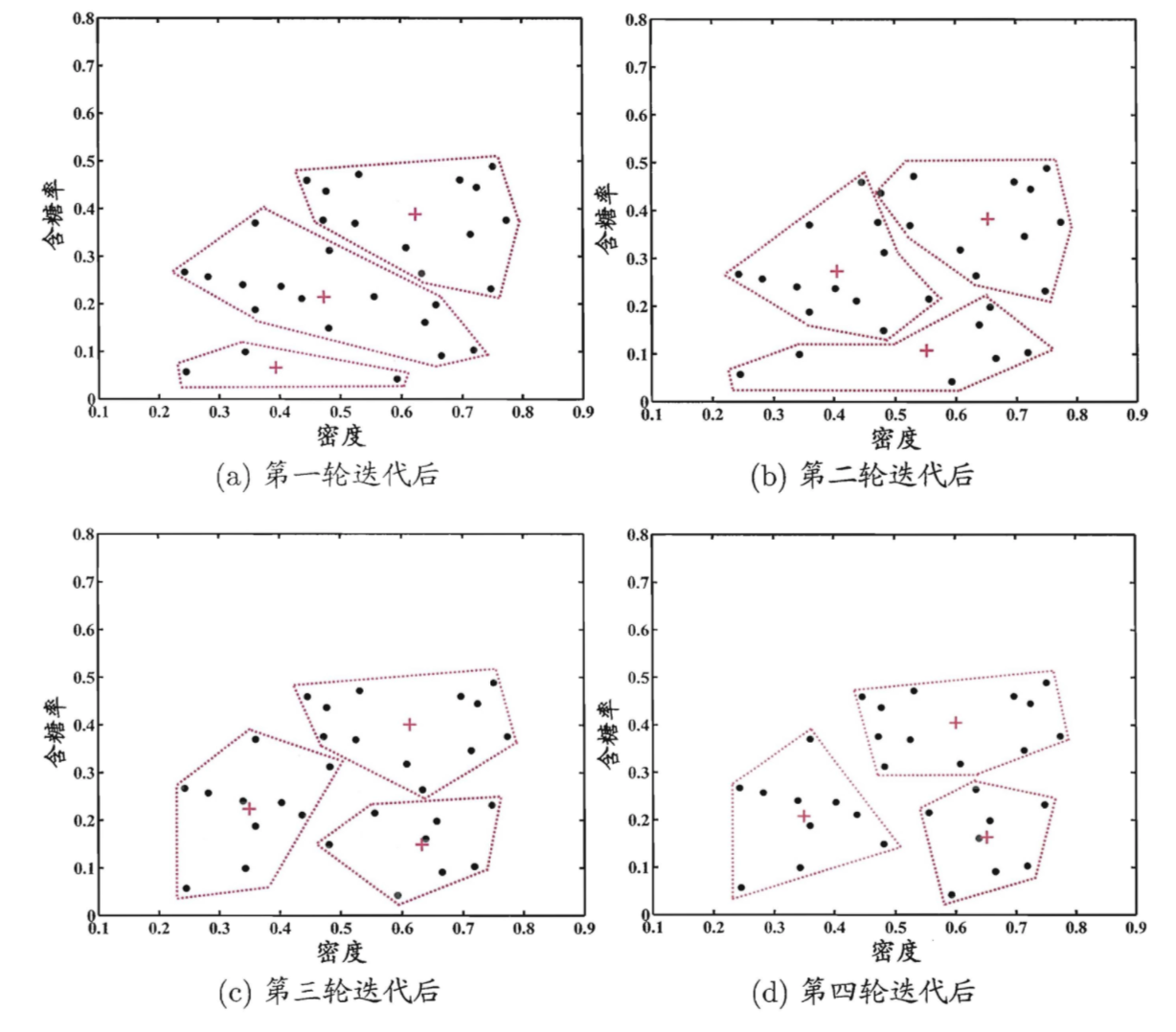图2 k 均值算法 4 轮迭代后的簇划分

标准 KMeans 的聚类结果受初始均值向量的影响，初始点不同，则聚类结果就有可能不同，因此可以通过多次随机初始化（n_init）聚类中心最终选取最优结果。
1.2 KMeans++
由于 KMeans 算法的分类结果会收到初始点的选取而有所区别，因此提出了标准 KMeans 的改进 KMeans++。
其改进在于对初始均值向量的选择，其他步骤同标准 KMeans 相同。初始均值向量选取的基本思路是：初始的聚类中心之间的相互距离要尽量远。
初始均值向量选取如下：
步骤一：随机选取一个样本作为第一个聚类中心 c1；
步骤二：
计算每个样本与当前已有类聚中心最短距离（即与最近一个聚类中心的距离），用 D(x)表示；  接着计算每个样本被选为下一个聚类中心的概率:

D

(

x

)

2

∑

x

∈

X

D

(

x

)

2

(2)

\frac{D(x)^2}{\sum_{x\in X}D(x)^2} \tag{2}

这个值越大，表示被选取作为聚类中心的概率较大；
最后，用轮盘法选出下一个聚类中心；
步骤三：重复步骤二，直到选出 k 个聚类中心。
1.2.1 KMeans++ 初始化实例
假设经过步骤一后 6 号点被选择为第一个初始聚类中心，那在进行步骤二时每个样本的

D

(

x

)

D(x)

和被选择为第二个聚类中心的概率

P

(

x

)

P(x)

如下表所示：

表2 第二个聚类中心的选择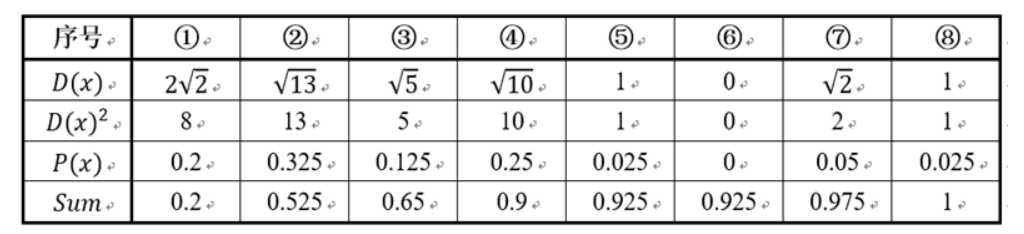其中的

P

(

x

)

P(x)

就是每个样本被选择为下一个聚类中心的概率。因此

P

(

x

)

P(x)

可以看作 PDF，Sum 可以看作 CDF，是

P

(

x

)

P(x)

的累加和，用于轮盘法选择出第二个聚类中心。
轮盘法方法就是随机产生一个 0～1 之间的随机数，判断随机数属于 Sum 行的哪个区间，那么该区间对应的序号就是被选择出来的第二个聚类中心了。例如 1 号点的区间为

[

0

,

0.2

)

[0,0.2)

，2 号点的区间为

[

0.2

,

0.525

)

[0.2,0.525)

。
从表中可以直观的看到第二个初始聚类中心有 90% 的概率落在 1～4 号点。而这 4 个点正好是离第一个聚类中心 6 号点较远的四个点。这也验证了 KMeans++ 的思想：即离当前已有聚类中心较远的点有更大的概率被选为下一个聚类中心。
当 k 值大于 2 时，每个样本会有多个距离，需要取最小的那个距离作为

D

(

x

)

D(x)

。
重复步骤 2 直到选出 k 个聚类中心，并利用这 k 个初始聚类中心来运行标准 KMeans 算法。
2. 在线可视化 KMeans
这个网站可以通过自己设定初始值方式以及数据分布，来进行迭代过程的可视化，有兴趣的也可以试试看。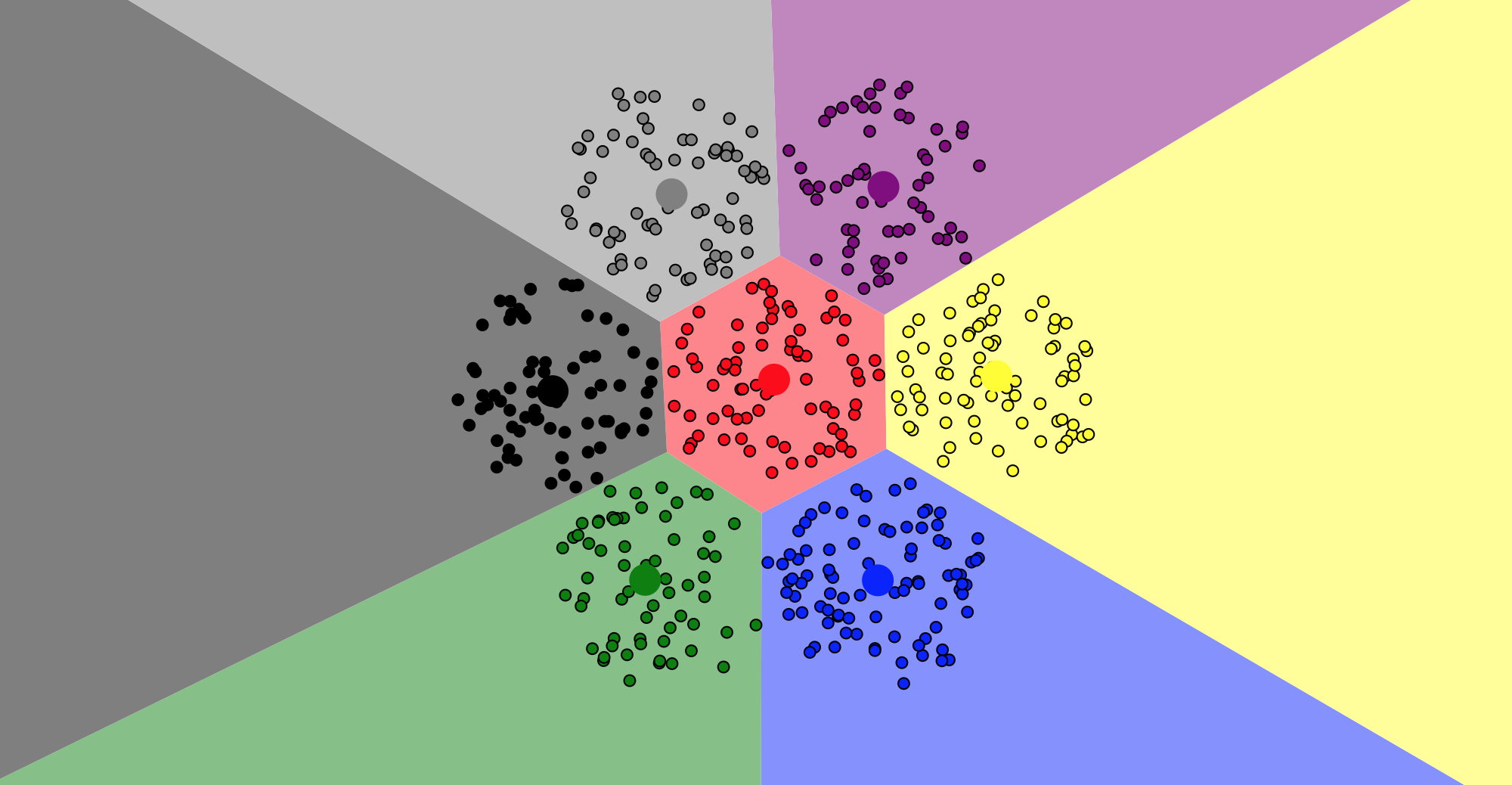参考资料
 周志华. 机器学习[M]. 北京: 清华大学出版社, 2016: 202-205.
 寒杰士.[ML] K-means与K-means++[EB/OL].https://www.cnblogs.com/wang2825/articles/8696830.html, 2018-04-02.
 0过把火0.[ML] K-means++[EB/OL].https://www.jianshu.com/p/680dbffad345, 2018-10-19.
展开全文机器学习 无监督学习 聚类算法
• birch，Kmeans，Kmeans++，KNN四种聚类算法对同一个二维坐标数据集进行聚类分析，python代码
• 最近学习了，Kmeans，Kmeans++，模糊Kmeans 看了几篇关于Kmeans的文献 首先讲解下各个算法的流程 Kmeans： ①随机选择K个对象作为初始的聚类中心 ②计算每个对象与各个聚类中心的距离 ③将对象分配给距离最近...python 聚类算法
• 基于Python3.7实现的KMeans++算法，包括源程序和测试数据。
• 自己用python手写实现了kmeans与kmeans++算法。记录一下，说不定以后就用着了呢。 首先是用到的几个自定义函数： def nearest(data,cluster_center): n = len(cluster_center) m = len(data) sum1 = 0 dis = [] ...kmeans
• 2、kmeans++因为，kmeans的初始种子的随机找的，这样，算法的收敛快慢与初始值关系非常大，于是，kmeans++ 主要针对初始值的选取进行改进。 初始值选取，如下：1、也是随机选取一个种子； 2、计算其他点到这算法
• 手写算法-python代码实现Kmeans++以及优化聚类结果不稳定的优化方法一次优化：kmeans++二次优化：添加参数n_init其他问题的优化方法 聚类结果不稳定的优化方法 上篇文章，我们列举了Kmeans的不足之处，也用python...聚类 python 机器学习
• 基于Python3.7实现KMeans++算法，并用于实现图像分割功能。包括源程序、测试图片、结果图片和运行步骤。
• Kmeans++、Mini-Batch Kmeans、Bisecting Kmeans、K中心点（K-Medoids）算法、K众数聚类、核K均值聚类.pdf
• 简述 在Kmeans当中，有两个限制 定义在凸欧式空间上，使得在非凸空间上的聚类效果一般，在非欧式空间上无法计算均值点。...KMeans++这篇论文主要关注于第二个问题。 KMeans的算法和实现就不再...算法 python
• 1. Kmeans++ Kmeans 中对聚类中心的初始化比较敏感，不同的初始值会带来不同的聚类结果，这是因为 Kmeans 仅仅是对目标函数求近似最优解，不能保证得到全局最优解。 在常规的 Kmeans 中，聚类中心的初始化都采用随机...
• 下面介绍Kmeans以及Kmeans++算法理论以及算法步骤： 根据样本特征选择不同的距离公式，程序实例中采用欧几里得距离。下面分别给出Kmeans以及Kmeans++算法的步骤。 Kmeans聚类算法的结果会因为初始的类别中心的...kmeans sklearn 聚类 机器学习
• estimator =KMeans(n_clusters=3,init = 'kmeans++') #构造一个聚类数为5的聚类器,初始质心选取方式改为kmeans++ estimator.fit(data1) #聚类 label_pred = estimator.labels_ #获取聚类标签 centroids = estimator....
• 1. Kmeans算法流程 随机初始化K个中心点； 计算所有样本分别到K个中心点的距离； 比较每个样本到K个中心点的距离（这里的距离也可以使用欧氏距离）...Kmeans++的算法思想是使得初始化的聚类中心点之间的距离尽可能的算法 聚类 机器学习 人工智能
• 标准K-means算法的缺陷、K-mean++初始化算法、初始化算法步骤、Kmeans++算法实现.pdf
• KMeans++ 的初始化方法： 1、随机选一个样本作为第一个簇中心。 2、计算每个样本到最近簇中心的距离。 3、以距离为概率随机选择下一个簇中心。 4、重复步骤2和3，直到找到K个簇中心。 vlfeat \ kmeans.c \ _vl_...
• K均值PP KMeans++ 的 AC# 实现。 比原始 KMeans 更准确。 它使用播种方法解决了 KMeans 的初始化问题，该方法使初始 k 个簇的中心彼此远离。 更多详情： : :C#
• 0 写在前面（数据集和源代码）本文章...一共有四个代码文件，分别是Kmeans、Kmeans++、Birch和KNN算法，四个算法对同一个数据集聚类分析进行对比试验。（本代码是本人自己书写，全部可用！）1 引言近年来，机器学习...机器学习 Kmeans聚类 Birch KNN聚类
• 一、K-Means使用 load_iris 二、KMeans++实现 三、KMeans基本框架实现 有趣的事，Python永远不会缺席 培训说明
• Kmeans++、Mini-Batch Kmeans、Bisecting Kmeans、K中心点（K-Medoids）算法、K众数聚类、核K均值聚类 目录 Kmeans++、Mini-Batch Kmeans、Bisecting Kmeans、K中心点（K-Medoids）算法、K众数聚类、核K均值聚类 ...人工智能 机器学习 聚类 数据挖掘 深度学习
• 我们知道Kmeans聚类算法在理论上有一定弊端，由于初始化完全随机，会使得生成的点收到限制，最后聚类的结果不好，且 kmeans算法由于初始“簇中心”点是随机...正因如此，Kmeans++作为Kmeans的衍生版本出现了 k-means...
• 1、从Kmeans说起 Kmeans是一个非常基础的聚类算法，使用了迭代的思想，关于其原理这里不说了。下面说一下如何在matlab中使用kmeans算法。 创建7个二维的数据点：复制代码 代码如下:x=[randn(3,2)*.4;randn(4,2)*.5+...
• kmeans算法存在的一个问题是初始中心的选取是随机的，造成聚类的结果也是...Kmeans++算法可以很好的解决初始点的选取问题，本文简单进行了总结和实现，并结合kmeans++算法提到了蓄水池算法在ClouderaML中的两个应用。聚类 算法 蓄水池采样...